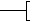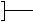Concepts from Chapters 32-36 inclusiveConcepts and associated formulas that you should know: Self-induced emf and its relation to the inductance; IR drop through a resistor; potential difference across a capacitor; relation between the induced emf in one coil, the mutual inductance, and the time rate of change of current in the other coil; how to solve the RLC circuit subject to initial conditions; relation between rms and max quantities for AC currents and voltages; various kinds of reactance, resistance, and impedance; resonance in a series RLC circuit; transformers; plane electromagnetic waves--velocity, wavelength, frequency, angular wave number, and angular frequency; index of refraction, rules for reflection and refraction; how to derive the condition for total internal reflection; real and virtual images; magnification; formation of images by mirrors, refractive surfaces and lenses; use of the lens equation; ray diagrams; conventions for when distances are positive or negative.Questions and/or comments should be sent to Oscar Greenberg. Last modified April 5, 2001       © 2004 University of Maryland University of Maryland   |   College Park, MD 20742   |   301.405.1000